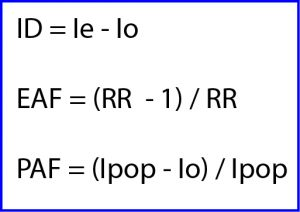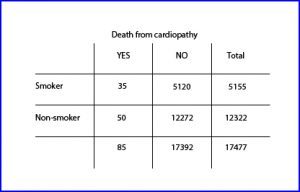Science without sense…double nonsense

Simplifying the impact

This post is also available in: Spanish

In epidemiological studies it is common to find a set of measures of effect such as risks in exposed and non-exposed, relative risks and risk reductions. However, in order for the analysis of a study to be considered well done, measures of effect should be accompanied by a series of impact measures, which are the ones that inform us more precisely about the true effect of the exposure or intervention we are studying.

For example, if we conducted a study on the prevention of mortality from a disease with a treatment X, a relative risk of 0.5 would tell us that there is a half chance of dying if we take the drug, but we cannot see clearly the impact of treatment. However, if we calculate the number needed to treat (NNT) and it comes out to be two, we will know that one in two people treated will avoid death by that disease. This impact measure, the NNT, does give us a clearer idea of the real effect of the intervention in our practice.

There are several impact measures, in addition to the NNT. In the cohort studies, which we are going to focus on today, we can calculate the difference of incidences between exposed and unexposed, the exposed attributable fraction (EAF), the avoidable risk in exposed (ARE) and the population attributable fraction (PAF).

The EAF indicates the risk of presenting the effect on the exposed that is due specifically to that, to have been exposed. The ARE would inform us of the cases of illness in the exposed group that could have been avoided had the exposure not existed. Finally, PAF is a specific attributable risk that describes the proportion of cases that could be prevented in the population if the risk factor under study were completely eliminated.As a fourth parameter, considering the presence of exposure and disease, we can calculate the fraction of exposure in cases (FEc), which defines the proportion of exposed cases that are attributable to the risk factor.

In the table that I attach you can see the formulas for the calculation of these parameters.

The problem with these impact measures is that they can sometimes be difficult to interpret on the part of the clinician. For this reason, and inspired by the calculation of NNTs, a series of measures called impact numbers have been devised, giving us a more direct idea of the effect of the exposure factor on the disease being studied. These impact numbers are the number of impact on exposed (NIE), the number of impact in cases (NIC) and the number of impact of exposed cases (NIEC).

Let’s start with the simplest. The NIE would be the equivalent of the NNT and would be calculated as the inverse of the absolute risk reduction or the risk difference. The NNT is the number of people who should be treated to prevent a case compared to the control group. The NIE represents the average number of people who have to be exposed to the risk factor for a new disease event to occur compared to non-exposed persons. For example, a NIE of 10 means that out of every 10 exposed a case of disease will occur that will be attributable to the risk factor.

The NIC is the inverse of the PAF, so it defines the average number of sick people among whom a case is due to the risk factor. A NIC of 10 means that for every 10 cases in the population, one is attributable to the risk factor under study.

Finally, the NIEC is the inverse of the FEc. It is the average number of cases among which a case is attributable to the risk factor.

In summary, these three measures indicate the impact of exposure among all exposed (NIE), among all patients (NIC) and among all patients who have been exposed (NIEC).An example is the data from the attached table, corresponding to a fictional study on the effect of coronary mortality on smoking. I have used an epidemiological calculator of the many available on the Internet and have calculated a risk difference of 0.0027, a PAR of 0.16 and an FEc of 0.4. We can now calculate our impact numbers.

NIE value is 1 / 0.0027 = 366. Rounding up, out of every 365 smokers, one will die from a heart disease attributable to tobacco.

NIC will be 1 / 0.16 = 6.25. Of every six deaths from heart disease in the population, one will be attributable to tobacco.

Finally, NIEC will be 1 / 0.4 = 2.5. Approximately, for every three deaths from heart disease among those who smoked, one would be attributable to tobacco addiction.

And here we leave it for today. Do not forget that the data of the example are fictitious and I do not know if they fit very much to reality.

We have discussed only the point estimates of impact numbers but, as always, it is preferable to calculate their confidence intervals. All three can be calculated with the limits of intervals of the measurement from which the impact numbers are obtained, but it is best to use a calculator that does it for us. Calculation of the intervals of some parameters such as, for example, the PAR can be complex. But that is another story…

This site uses Akismet to reduce spam. Learn how your comment data is processed.